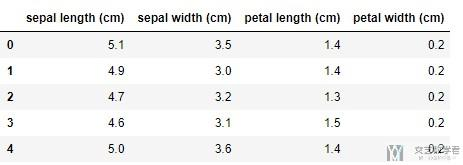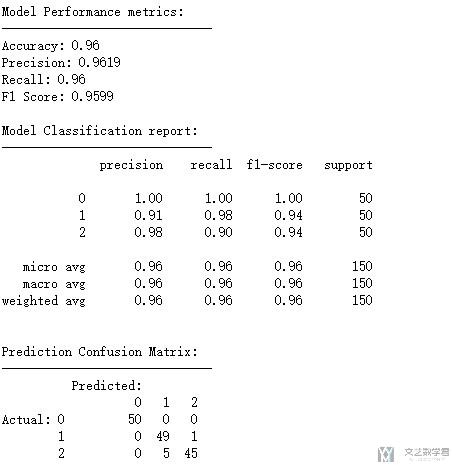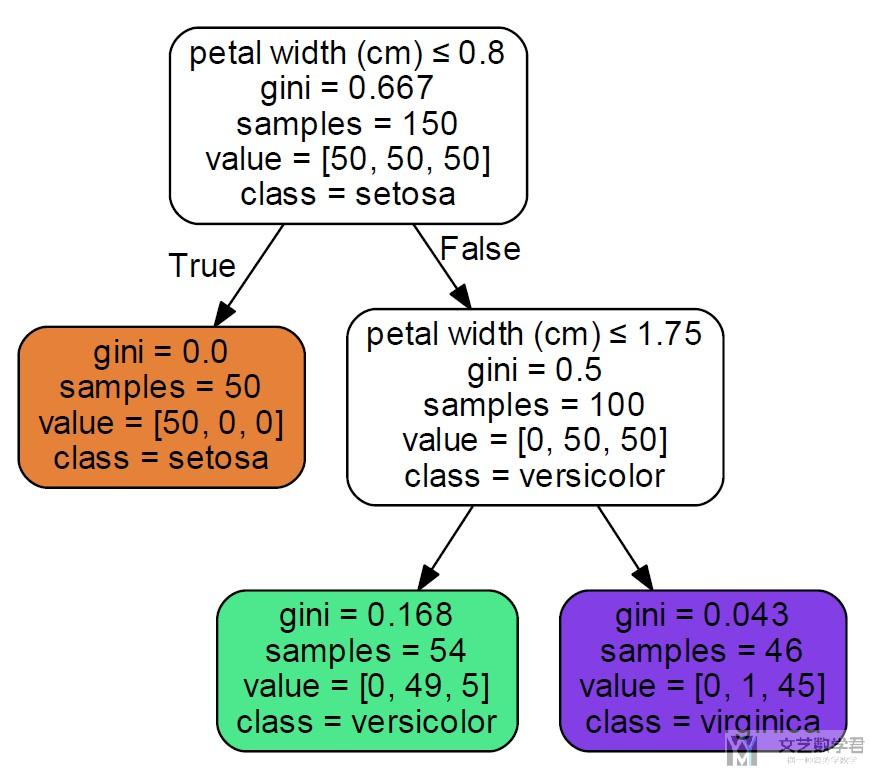# 决策树案例(Decision Tree)与决策树的可视化2019年10月19日07:27:39

2617字阅读8分43秒

## 模型的训练与可视化

### 导入数据集

1. import sklearn.datasets as datasets
2. import pandas as pd

2. df = pd.DataFrame(data=iris.data, columns=iris.feature_names)
3. y = iris.target### 训练决策树模型

1. from sklearn.tree import DecisionTreeClassifier
2. # 训练决策树模型(控制决策树的深度, 这里控制最大深度是2)
3. dtree = DecisionTreeClassifier(max_depth=2)
4. dtree.fit(df, y)
5. """
6. DecisionTreeClassifier(class_weight=None, criterion='gini', max_depth=2,
7.             max_features=None, max_leaf_nodes=None,
8.             min_impurity_decrease=0.0, min_impurity_split=None,
9.             min_samples_leaf=1, min_samples_split=2,
10.             min_weight_fraction_leaf=0.0, presort=False, random_state=None,
11.             splitter='best')
12. """

### 模型的评价

1. from tools import *

1. # 模型的评估
2. y_pre = dtree.predict(df)
3. display_model_performance_metrics(true_labels=y,
4.                              predicted_labels=y_pre,
5.                                  classes=[0,1,2])### 结果的可视化

1. from sklearn.tree import export_graphviz
2. import graphviz

1. import os
2. os.environ["PATH"] += os.pathsep + 'C:/Program Files (x86)/Graphviz2.38/bin'

1. dot_data = export_graphviz(dtree, out_file=None,
2.                            feature_names=iris.feature_names,
3.                            class_names=iris.target_names,
4.                            filled=True, rounded=True,
5.                            special_characters=True)
6. # 可以设置图像的显示
7. graph = graphviz.Source(dot_data)

1. graph.render(filename ="iris", directory ='./', format='pdf')## 一些问题

### Make sure the Graphviz executables are on your systems’ PATH

1. RuntimeError: failed to execute ['dot', '-Tpdf', '-O', 'test'], make sure the Graphviz executables are on your systems' path

1. import os
2. os.environ["PATH"] += os.pathsep + 'C:/Program Files (x86)/Graphviz2.38/bin'

• 微信公众号
• 关注微信公众号
•• QQ群
• 我们的QQ群号
•• 本文由 发表于 2019年10月19日07:27:39
• 转载请务必保留本文链接：https://mathpretty.com/11308.html